# JEE Main 2020 Chemistry Paper With Solutions Sept 6 (Shift 1)

JEE Main 2020 Shift 1- Sept 6th Chemistry solved questions are available at BYJU’S. Students can also download these solutions from our website in PDF format. Practising sample question papers help the candidates to get an idea of the weightage given to various topics, solve questions faster and learn time management. It also helps to understand the question paper pattern and to get an idea about the questions frequently asked in the examination.

### September 6 Shift 1 - Chemistry

1. The INCORRECT statement is:

1. 1) Cast iron is used to manufacture wrought iron.
2. 2) Brass is an alloy of copper and nickel.
3. 3) German silver is an alloy of zinc, copper and nickel.
4. 4) Bronze is an alloy of copper and tin

Solution:

Brass - (copper Zinc)

Bronze - (copper tin)

2. The species that has a spin-only magnetic moment of 5.9 BM, is: (Td= tetrahedral)

1. 1) [Ni(CN)4]2- (square planar)
2. 2) Ni(CO)4(Td)
3. 3) [MnBr4]2-(Td)
4. 4) [NiCl4]2-(Td)

Solution:

[MnBr4]2–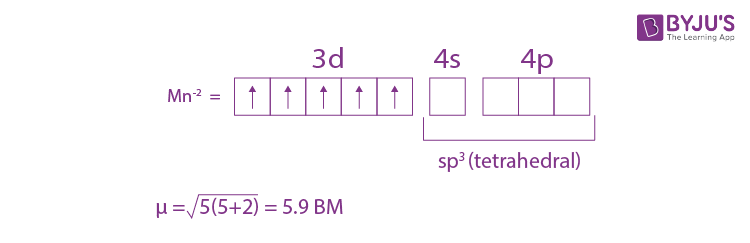3. For the reaction

$$Fe_{2}N(s)+\frac{3}{2}H_{2}(g)\rightleftharpoons 2Fe(s)+NH_{3}(g)$$

1. 1) Kc= Kp(RT)1/2
2. 2) Kc= Kp(RT)-1/2
3. 3) Kc= Kp(RT)3/2
4. 4) Kc= Kp(RT)

Solution:

$$Fe_{2}N(s)+\frac{3}{2}H_{2}(g)\rightleftharpoons 2Fe(s)+NH_{3}(g)$$

Δng =1-3/2 = -1/2

(Kp/Kc)= (RT)Δng = (RT)-1/2

Kc= Kp/(RT)-1/2 = Kp.(RT)1/2

4. Consider the following reactions: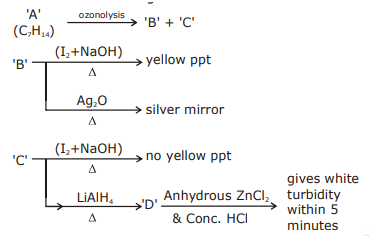'A' is:

Solution:

1.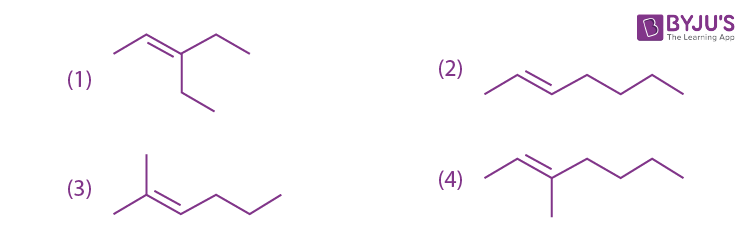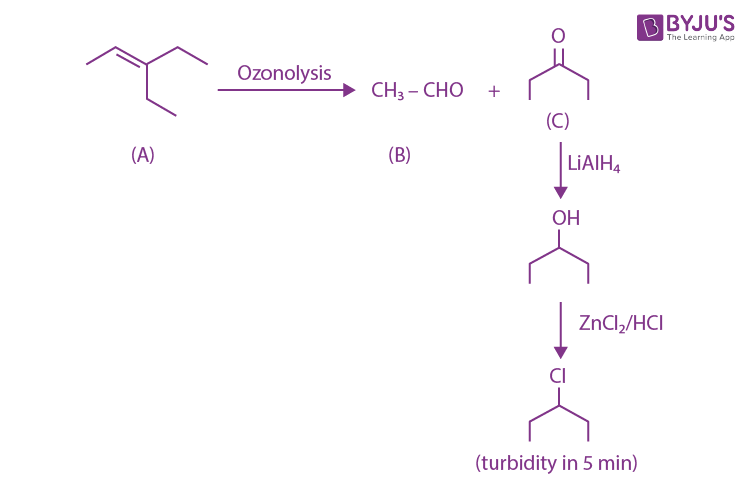5. Arrange the following solutions in the decreasing order of pOH:

(A) 0.01 M HCl

(B) 0.01 M NaOH

(C) 0.01 M CH3COONa

(D) 0.01 M NaCl

1. 1) (A) > (C) > (D) > (B)
2. 2) (B) > (D) > (C) > (A)
3. 3) (B) > (C) > (D) > (A)
4. 4) (A) > (D) > (C) > (B)

Solution:

(i) 10–2 M HCl ⇒ [H+] = 10–2 M → pH = 2

(ii) 10–2 M NaOH ⇒ [OH–] = 10–2 M → pOH = 2

(iii) 10–2 M CH3COONa+⇒ [OH+] > 10–7 ⇒ pOH < 7

(iv) 10–2 M NaCl ⇒ Neutral pOH = 7

(i) > (iv) > (iii) > (ii)

6. The variation of equilibrium constant with temperature is given below :

Temperature Equilibrium Constant

T1= 250C K1= 10

T2= 1000C K2= 100

The value of ∆H0 , ∆G0 at T1 and ∆G0 at T2 (in Kj mol-1) respectively, are close to

[use R = 8.314JK-1 mol-1]

1. 1) 28.4, -7.14 and -5.71
2. 2) 0.64, - 7.14 and -5.71
3. 3) 28.4, - 5.71 and -14.29
4. 4) 0.64, - 5.71 and -14.29

Solution:

$$In\frac{k_{2}}{k_{1}}=\frac{\Delta H^{0}}{R}\left \{ \frac{1}{T_{1}}-\frac{1}{T_{2}} \right \}$$

$$In(10)=\frac{\Delta H^{0}}{R}\left \{ \frac{1}{298}-\frac{1}{373} \right \}$$

$$\frac{373\times 298\times 8.314\times 2.303}{75}=\Delta H^{0}=28.37 kjmol^{-1}$$

∆G0T1 = –RT1ln(K1) = –298R ln(10) = –5.71 kJ mol–1

∆G0T2= –RT2ln(K2) = –373R ln(100)

= –14.283 kJ/mol

7. Consider the following reactions

A→ P1; B→ P2; C→ P3; D →P4,

The order of the above reactions are a,b,c and d, respectively. The following graph is obtained when log[rate] vs. log[conc.] are plotted.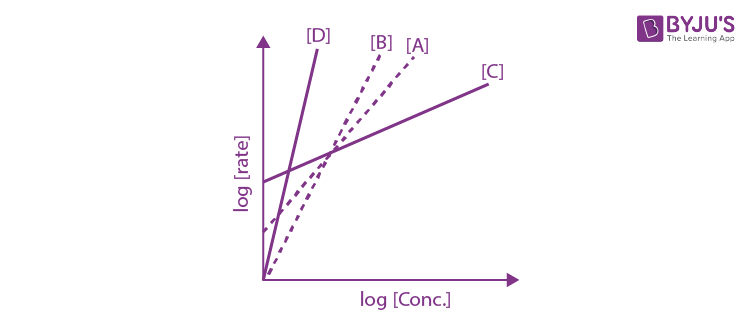Among the following the correct sequence for the order of the reactions is:

1. 1) c > a > b > d
2. 2) d > a > b > c
3. 3) d > b > a > c
4. 4) a > b > c > d

Solution:

A→ P1; B→ P2; C→ P3; D →P4,

Rate= K(conc)order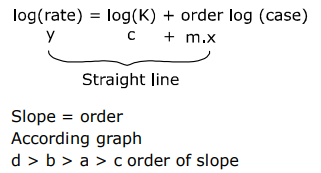8. The major product obtained from the following reactions is:

Solution:

1.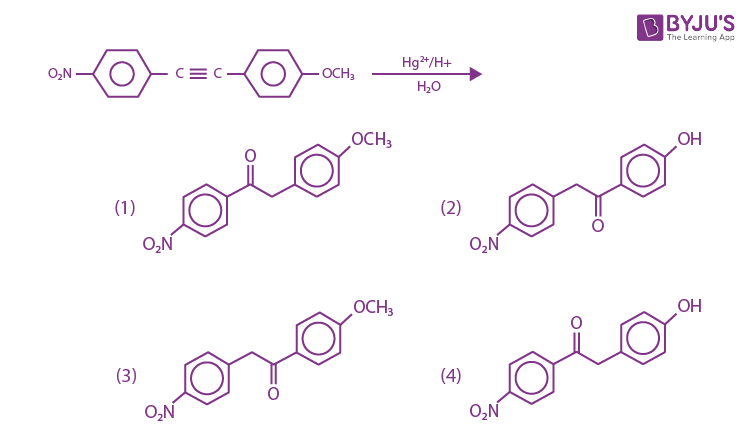Solution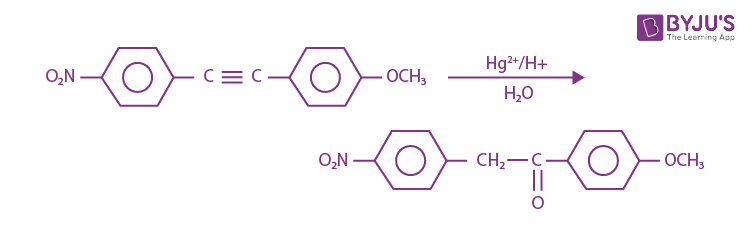9. Which of the following compounds shows geometrical isomerism?

1. 1) 2-methylpent-1-ene
2. 2) 4-methylpent-2-ene
3. 3) 2-methylpent-2-ene
4. 4) 4-methylpent-1-ene

Solution:

1.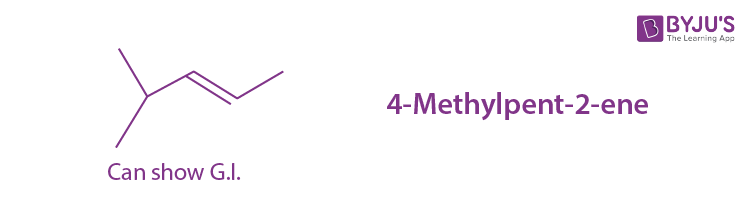10. The lanthanoid that does NOT shows +4 oxidation state is:

1. 1) Dy
2. 2) Ce
3. 3) Tb
4. 4) Eu

Solution:

Fact

11. The major products of the following reactions are:

Solution:

1.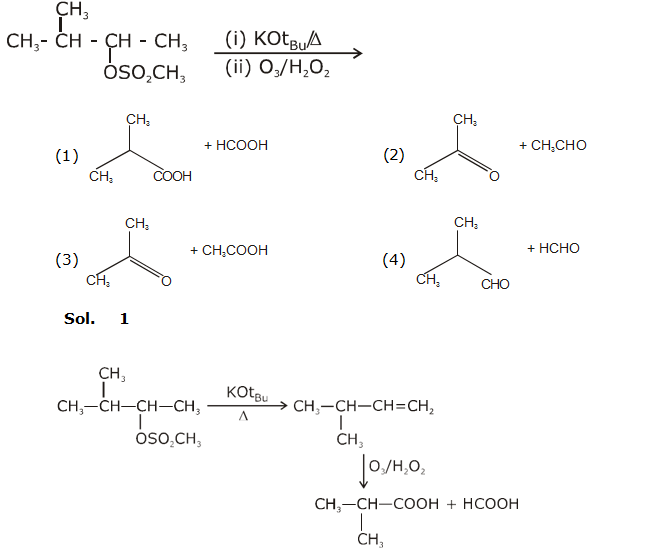12. The major product of the following reaction is:

Solution:

1.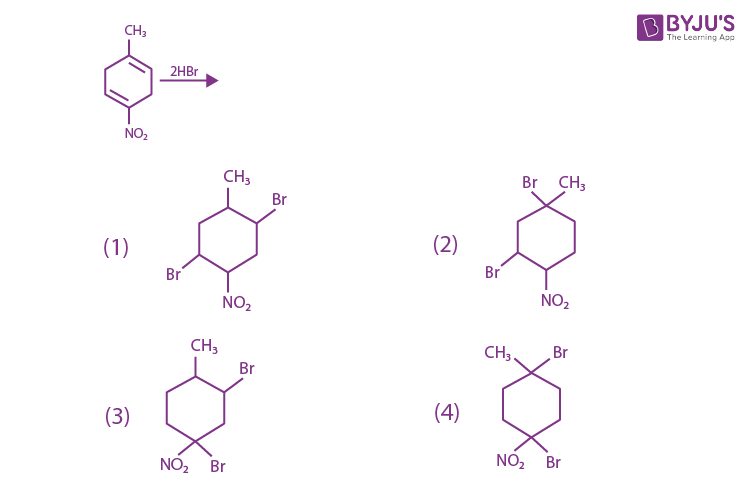2.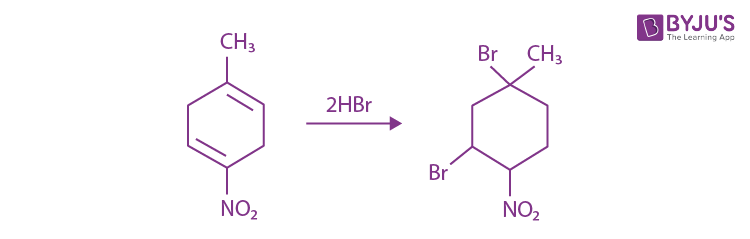13. The increasing order of pKb values of the following compounds is:

Solution:

1.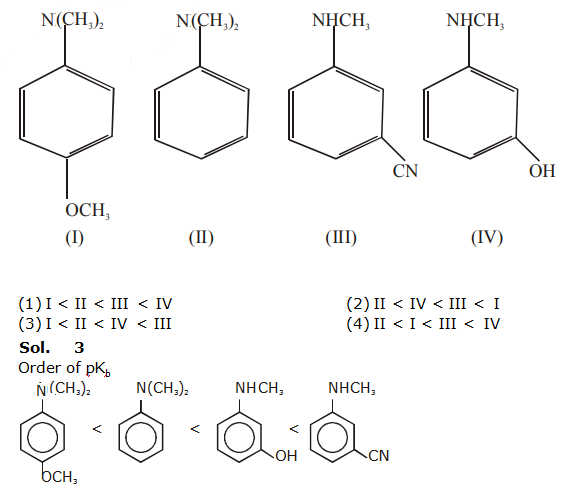14. kraft temperature is the temperature :

1. 1) Above which the aqueous solution of detergents starts boiling
2. 2) Below which the formation of micelles takes place.
3. 3) Above which the formation of micelles takes place.
4. 4) Below which the aqueous solution of detergents starts freezing.

Solution:

TK+ temp. above which formation of micelles takes place.

15. The set that contains atomic numbers of only transition elements, is?

1. 1) 9, 17, 34, 38
2. 2) 21, 25, 42, 72
3. 3) 37, 42, 50, 64
4. 4) 21, 32, 53, 64

Solution:

Tranition elements = 21 to 30

37 to 48

57 & 72 to 80

Ans. 21, 25, 42 & 72

16. Consider the Assertion and Reason given below.

Assertion (A): Ethene polymerized in the presence of Ziegler Natta Catalyst at high temperature and pressure is used to make buckets and dustbins.

Reason (R): High density polymers are closely packed and are chemically inert. Choose the correct answer from the following:

1. 1) (A) and (R) both are wrong.
2. 2) Both (A) and (R) are correct and (R) is the correct explanation of (A)
3. 3) (A) is correct but (R) is wrong
4. 4) Both (A) and (R) are correct but (R) is not the correct explanation of (A)

Solution:

From ziegler - Natta catalyst HDPE is produced, HDPE is closely packed and are chemically inert, so used to make backet and dust.

17. A solution of two components containing n1 moles of the 1st component and n2 moles of the 2nd component is prepared. M1 and M2 are the molecular weights of component 1 and 2 respectively. If d is the density of the solution in g mL-1, C2 is the molarity and x2 is the mole fraction of the 2nd component, then C2 can be expressed as:

1. 1)
$$C_{2}=\frac{dx_{1}}{M_{2}+x_{2}(M_{2}-M_{1})}$$
2. 2)
$$C_{2}=\frac{1000x_{2}}{M_{1}+x_{2}(M_{2}-M_{1})}$$
3. 3)
$$C_{2}=\frac{dx_{2}}{M_{2}+x_{2}(M_{2}-M_{1})}$$
4. 4)
$$C_{2}=\frac{1000dx_{2}}{M_{1}+x_{2}(M_{2}-M_{1})}$$

Solution:

$$C_{2}=\frac{x_{2}}{\left [ x_{_{2}}M_{1}+(1-x_{2})(M_{2}) \right ]/d}\times 1000$$

$$C_{2}=\frac{1000dx_{2}}{M_{1}+x_{2}(M_{2}-M_{1})}$$

18. The correct statement with respect to dinitrogen is?

1. 1) Liquid dinitrogen is not used in cryosurgery.
2. 2) N2 is paramagnetic in nature
3. 3) It can combine with dioxygen at 250 C
4. 4) It can be used as an inert diluent for reactive chemicals.

Solution:

(1) Liquid nitrogen is used as a refrigerant to preserve biological material food items and in cryosurgery.

(2) N2 is diamagnetic, with no unpaired elctrons.

(3) N2 does not combine with oxygen, hydrogen or most other elements. Nitrogen will combine with oxygen, however; in the presence of lightining or a spark.

(4) In iron and chemical Industry inert diluent for reactive chemicals.

19. Among the sulphates of alkaline earth metals, the solubility of BeSO4 and MgSO4 in water, respectively, are:

1. 1) Poor and high
2. 2) High and high
3. 3) Poor and poor
4. 4) High and poor

Solution:

Order of solubility of sulphate of Alkaline earth metals

BeSO4> MgSO4 > CaSO4 > SrSO4> BaSO4

20. The presence of soluble fluoride ion upto 1ppm concentration in drinking water, is:

1. 1) Harmful to skin
2. 2) Harmful to bones
3. 3) Safe for teeth
4. 4) Harmful for teeth

Solution:

Environmental chemistry - safe for teeth

21. A spherical balloon of radius 3cm containing helium gas has a pressure of 48 × 10-3 bar. At the same temperature, the pressure, of a spherical balloon of radius 12cm containing the same amount of gas will be.........................× 10-6 bar.

Solution:

$$moles = \frac{48\times 10^{-3}\times \frac{4}{3\pi }(3cm)^{3}}{R\times T}$$

$$moles = \frac{P\times \frac{4}{3\pi }(12cm)^{3}}{R\times T}$$

P × 144 × 12 = 48 × 9 × 3 × 10–3

P = (27/36) x 10-3

P = 750 × 10–6 bar

22. The elevation of boiling point of 0.10m aqueous CrCl3 xNH3 solution is two times that of 0.05 m aqueous CaCl2 solution. The value of x is.................

[Assume 100% ionisation of the complex and CaCl2, coordination number of Cr as 6, and that all NH3 molecules are present inside the coordination sphere]

Solution:

∆Tb = i × Kb × m

i × 0.1 × Kb = 3 × 0.05 × Kb × 2

i = 3

[Cr(NH3)5. Cl] Cl2 →[Cr(NH3)5Cl]+2 + 2Cl

x = 5

23. Potassium chlorate is prepared by the electrolysis of KCl in basic solution

6OH + Cl -> ClO3 + 3H2O + 6e

If only 60% of the current is utilized in the reaction, the time (rounded to the nearesthour) required to produce 10g of KClO3 using a current of 2A is .............

(Given: F = 96,500 C mol-1; molar mass of KClO3=122g mol-1)

Solution:

$$\frac{10}{122}\times 6 = \frac{2\times t(hr)\times 3600\times 60/100}{96500}$$

t(hr) =

$$t(hr)=\frac{96500}{122\times 72}=10.98 hr$$

= 11 hours

24. In an estimation of bromine by Carius method, 1.6 g of an organic compound gave 1.88 g of AgBr. The mass percentage of bromine in the compound is....... .(Atomic mass, Ag=108, Br=80 g mol–1)

Solution:

Carius method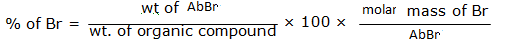= (1.88/1.6) x (80/188) x 100 = 15040/300.8 = 50 %

25. The number of Cl = O bonds in perchloric acid is, "..............."

Solution:### JEE Main 2020 Chemistry Paper With Solutions Shift 1 September 6